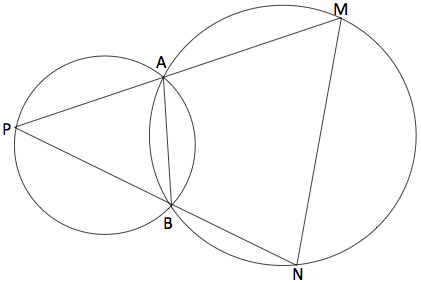SEARCH HOMEMath Central Quandaries & QueriesQuestion from Luke, a student: Two fixed circles intersect at A and B. P is a variable point on one circle. PA and PB when produced meet the other circle at M and N respectively. Prove that MN is of constant length. Thanks!Here is the diagram that luke sent.Hi Luke,

You just have to put together three familiar circle theorems:

1. If O is the center of your circle ABNM and P is outside, then angle NPM = (1/2)(angle NOM - angle AOB).

2. If O is the center of your circle ABNM and P is inside, then angle NPM = (1/2)(angle NOM + angle AOB).

3. (Using neutral letters for this theorem because you will have to apply it to both your circles) if X,Y,and Z are three points on a circle whose center is C, then when X and C are on the same side of the line YZ, angle YXZ = (1/2) angle YCZ; when on opposite sides, angle YXZ + (1/2) angle YCZ = 180 degrees.

ChrisMath Central is supported by the University of Regina and The Pacific Institute for the Mathematical Sciences.